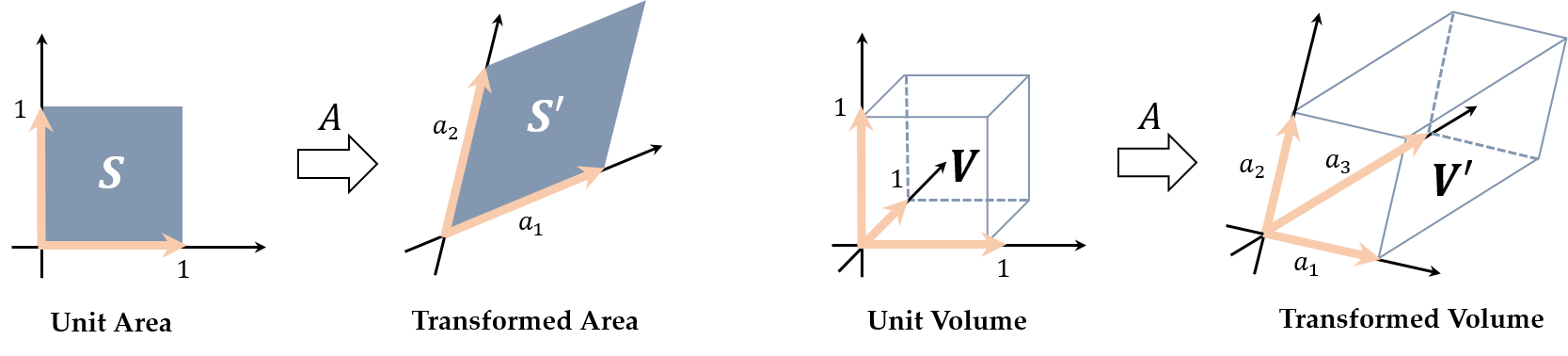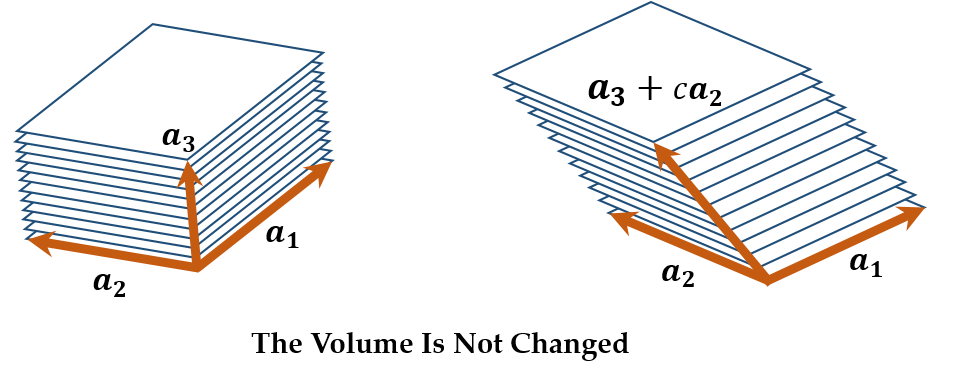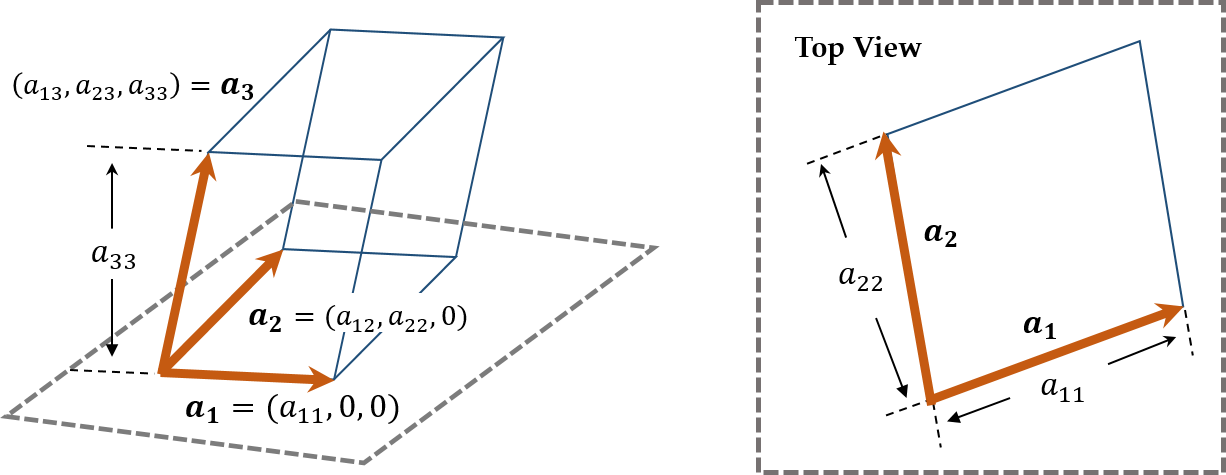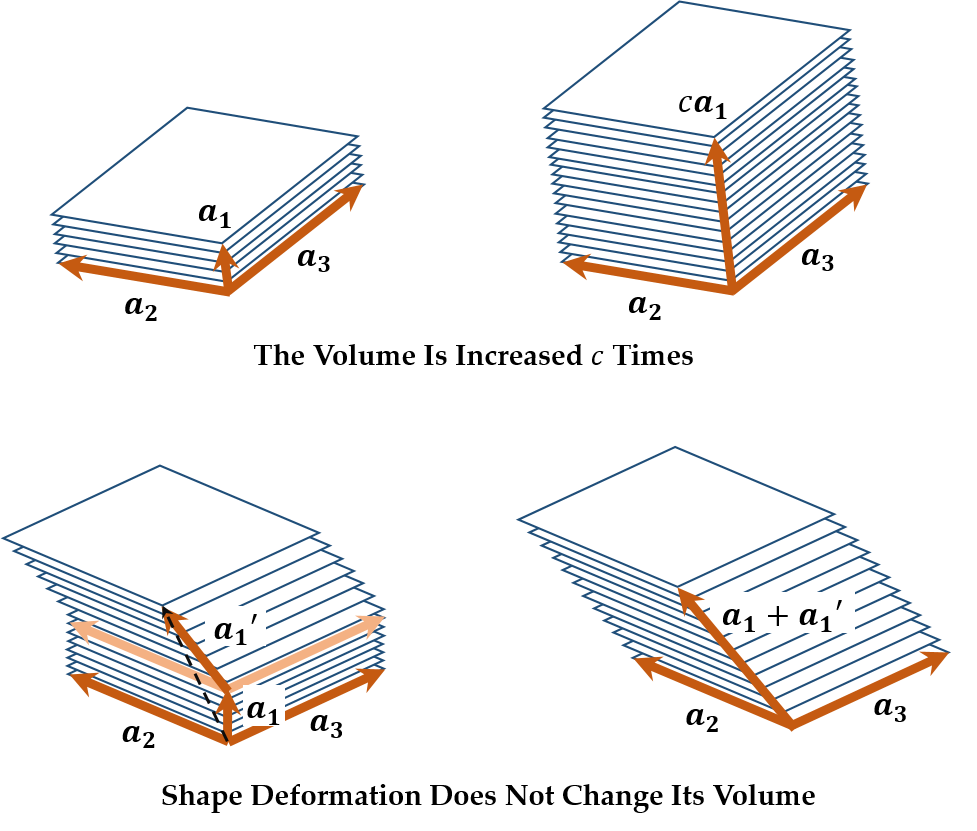# Determinant

• For an $n \times n$ matrix $A$, the determinant of $A$ denotes the magnification of the volume of $n$-dimensional parallelopiped.
• More formally, the following are the determinants of $2 \times 2$ and $3 \times 3$ matrices.If $A = (a_1, a_2)$ is a $2 \times 2$ matrix, $\det A$ means the transformed area from the unit area. If $A = (a_1, a_2, a_3)$ is a $3 \times 3$ matrix, $\det A$ means the transformed volume form the unit volume.

• For the arbitrary column vector $a_i$ of $A$, adding $ka_i$ to another column vector $a_j$ does not change $\det A$. For example, $\det (a_1, a_2, a_3) = \det (a_1, a_2, a_3 + ca_2)$ for $c \in \R$. Consider $\det A$ as a deck, then its volume is not changed although it is pushed to some directions.• The determinant of an upper triangular matrix $A$ is the product of all the diagonal elements of $A$.
\begin{aligned} A &= \left(\begin{array}{ccc} a_{11} & a_{12} & a_{13} \\ 0 & a_{22} & a_{23} \\ 0 & 0 & a_{33} \end{array}\right) \implies \det A = a_{11}a_{22}a_{33} \end{aligned}• For $A = (a_1, \cdots, a_n)$ and $c \in \R$, $\det (ca_1, a_2, \cdots, a_n) = c \det (a_1, \cdots, a_n)$. Moreover, $\det (a_1 + a'_1, a_2, \cdots, a_n) = \det (a_1, a_2, \cdots, a_n) + \det (a'_1, a_2, \cdots, a_n)$ for an arbitrary $n \times 1$ vector $a'_1$.## Reference

 Hiraoka Kazuyuki, Hori Gen, Programming No Tame No Senkei Daisu, Ohmsha.